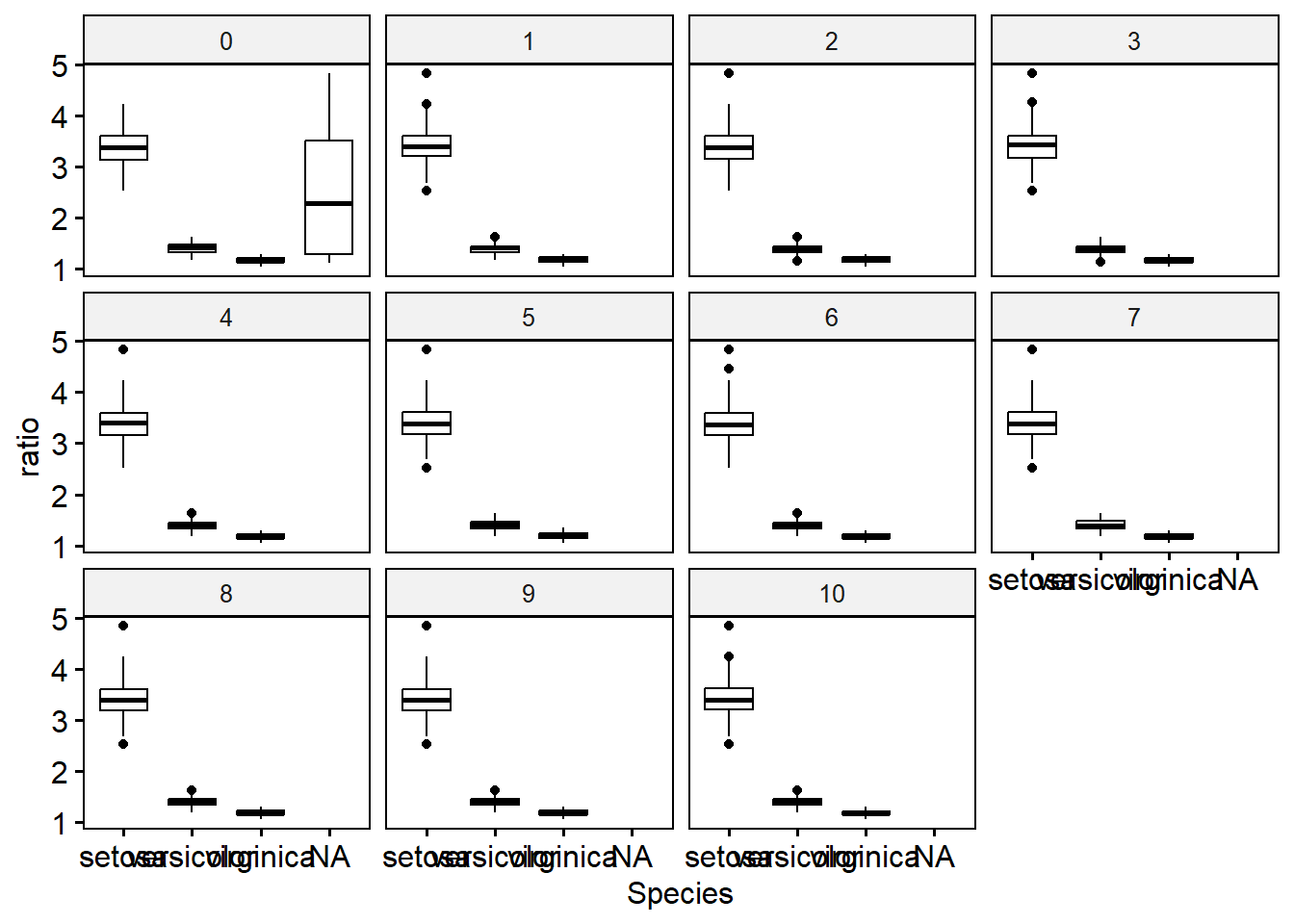## 18.10 Post MICE data management

Sometimes you’ll have a need to do additional data management after imputation has been completed. Creating binary indicators of an event, re-creating scale variables etc. The general approach is to transform the imputed data into long format using complete with the argument include=TRUE , do the necessary data management, and then convert it back to a mids object type.

Continuing with the iris example, let’s create a new variable that is the ratio of Sepal to Petal length.

Recapping prior steps of imputing, and then creating the completed long data set.

## imp_iris <- mice(iris.mis, m=10, maxit=25, meth="pmm", seed=500, printFlag=FALSE)
iris_long <- complete(imp_iris, 'long', include=TRUE)

We create the new ratio variable on the long data:

iris_long$ratio <- iris_long$Sepal.Length / iris_long\$Petal.Length

Let’s visualize this to see how different the distributions are across imputation. Notice imputation “0” still has missing data - this is a result of using include = TRUE and keeping the original data as part of the iris_long data.

ggpubr::ggboxplot(iris_long, y="ratio", x="Species", facet.by = ".imp")Then convert the data back to mids object, specifying the variable name that identifies the imputation number.

imp_iris1 <- as.mids(iris_long, .imp = ".imp")

Now we can conduct analyses such as an ANOVA (in linear model form) to see if this ratio differs significantly across the species.

nova.ratio <- with(imp_iris1, lm(ratio ~ Species))
pool(nova.ratio) |> summary()
##                term  estimate  std.error statistic       df       p.value
## 1       (Intercept)  3.439557 0.03597499  95.60967 105.1753 4.559615e-104
## 2 Speciesversicolor -2.048535 0.04990110 -41.05191 117.2154  2.090733e-71
## 3  Speciesvirginica -2.258591 0.05000935 -45.16337 119.3221  7.055165e-77